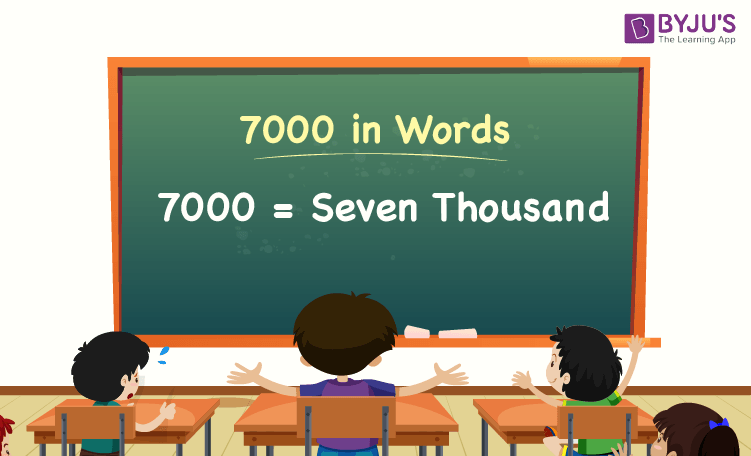# 7000 in Words

7000 in words is Seven Thousand. The number name of any number can be written using the ones, tens, hundreds, and thousands place of a number. Thus, the place value chart is helpful for writing the number 7000 in words. For example, if you spent Rs. 7000 in a month, you can write, “I have spent Rs. Seven Thousand in a month”.

 7000 in Words: Seven Thousand

In this article, let us discuss how to write 7000 in words, and look at the solved examples in detail.

## How to Write 7000 in Words?To write the number name of 7000, identify the place value of each digit of the given number 7000. Hence, for the number 7000, the digit in one’s place is 0, the digit in ten’s place is 0, the digit in hundred’s place is 0, and the number in thousand’s place is 7.

i.e.,1’s place of 7000 is 0.

10’s place of 7000 is 0.

100’s place of 7000 is 0.

1000’s place of 7000 is 7.

Thus, the place value chart for 7000 is:

 Thousands Hundreds Tens Ones 7 0 0 0

Therefore, the number 7000 in words is Seven Thousand.

### Examples

Example 1:

Find the value of 12000 – 5000. Express the value in words.

Solution:

Given expression: 12000 – 5000

⇒ 12000 – 5000 = 7000

So, the value of 12000 – 5000 is 7000.

Hence, 7000 in words is seven thousand.

Example 2:

Express the value of seven thousand plus ten thousand in words.

Solution:

Seven thousand = 7000

Ten thousand = 10000

Seven thousand plus ten thousand = 7000 + 10000 = 17000

Hence, the value of seven thousand plus ten thousand is seventeen thousand in words.

## Frequently Asked Questions on 7000 in Words

Q1

### Write 7000 in words.

7000 in words is seven thousand.

Q2

### Express the value of 6500 + 500 in words.

Simplifying 6500 + 500, we get 7000. Hence, 7000 in words is seven thousand.

Q3

### How to write seven thousand in numbers?

Seven thousand in numbers is 7000.

Q4

### Is the number 7000 an odd number?

No, the number 7000 is not an odd number.

Q5

### Is 7000 a composite number?

Yes, 7000 is a composite number.